This article was published in the C/C++ Users Journal in a two-part format. The first part of the article appeared in the November 1998 issue, and the second part in the December 1998 issue.

I hope you find it useful, didactic, or at the very least, I hope you enjoy reading it. You are free to use the code presented in this article, being understood that I decline any responsibility, direct or implied, for damages or inconveniences that its use or impossibility of use may cause (directly or indirectly). If your local law does not allow a full decline of responsibility by the author of a software that you use, then you are not allowed to use the code presented in this article or any part of it (neither from this web-page, nor downloaded from the C++ Users Journal web-site).

The opening line and the figure appearing with the title of the article are courtesy of the C++ Users Journal.

If you have any comments or question concerning the content or form, you can reach me by e-mail (visit my contact page for instructions)

# Efficient 2-D Geometric Operations

### Carlos Moreno

"Inside" is an easy predicate for people to determine, but rather harder for computers.

## Introduction

Geometric algorithms and techniques are important tools in Graphics, Image Processing and Computer Vision applications. They also offer convenient and efficient solutions to Pattern Recognition problems such as the Nearest Neighbor rules, Clustering, and Image or Polygons pre-processing.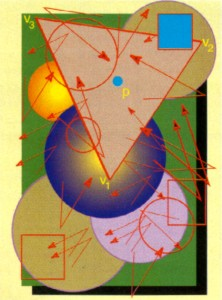In this article, I present a C++ implementation of some standard and very efficient algorithms and techniques in the field of Computational Geometry. This implementation includes fundamental operations such as orientation, test for point inclusion in a triangle, and segment intersection. These fundamental operations provide a basis for more complex operations, such as polygon orientation, point inclusion in a polygon, triangulation, convex hull computation for a set of points and for a simple polygon, and other standard algorithms.

The tools are based on the implementation of four classes, to represent points, segments, triangles and polygons, all of them in the plane (two-dimensional).

## The Mathematics of 2-D Operations

The basic operation at the heart of the algorithms presented is computation of a triangle's area given its three vertices (i.e., given the x-y coordinates of those vertices). This computation is efficiently performed based on the properties of the vector product.

Given two vectors v1,v2, their vector product v1 x v2 is perpendicular to both vectors; its magnitude is equal to the product of the magnitudes of v1 and v2 times the sine of the angle between them; and its orientation can be obtained using the Right Hand Rule.

If the two vectors are in the x-y plane (i.e., their z-coordinates are zero), their vector product will be parallel to the z-axis, and its z-coordinate will be positive if vector v2 is "at the left" of vector v1, zero if v1 and v2 are parallel, and negative if v2 is "at the right" of v1.

Given three points p1,p2,p3 in the x-y plane, the z-coordinate of the vector product between p2-p1 (p2 minus p1) and p3-p2 is given by the following formula:

z = x1 (y2 - y3) + x2 (y3 - y1) + x3 (y1 - y2)

 Given the properties just discussed for the vector product, the above formula yields the following information (see figure 1): The magnitude of z is twice the area of the triangle p1,p2,p3.  The sign of z tells whether the triplet p1,p2,p3 represents a right-turn or left-turn (that is, if the point p3 is at the right or at the left of the oriented segment from p1 to p2)  Notice that only three real number multiplications and five additions are required to compute this value. No trigonometric function calculations are involved.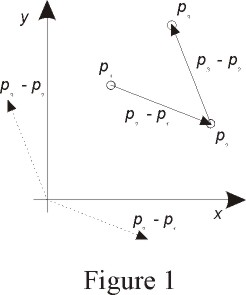Surprisingly enough, this simple operation forms the basis of most of the algorithms that I present in this article, as well as many other extremely complex algorithms. Even more surprising is the fact that what is (almost exclusively) used in the algorithms is the sign of Z (i.e., the orientation of triplets of points), rather than the magnitude or the value itself.

In addition to this fundamental operation and the basic arithmetic (including the scalar product), two other basic operations (both based exclusively on the orientation of triplets of points) complete the basis for all the algorithms that I present: a test for segment intersection, and a test for point inclusion in a triangle. These two operations are also based exclusively on the orientation of triplets of points.

### Testing for Segment Intersection

Given two segments (p1,p2) and (q1,q2), they intersect if and only if the orientation of the triplet (p1,p2,q1) is different from the orientation of the triplet (p1,p2,q2) and the orientation of the triplet (q1,q2,p1) is different from the orientation of the triplet (q1,q2,p2). The first condition means that q1 is on one side of the segment (p1,p2), and q2 is on the other side. The second condition means that p1 is on one side of the segment (q1,q2), and p2 is on the other side. Clearly, the segments intersect if and only if both conditions are met, as illustrated in figures 2-a and 2-b.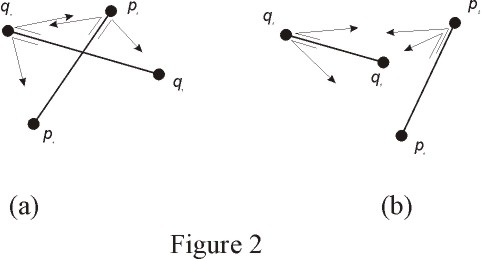### Testing for Point Inclusion in a Triangle

Given a triangle (v1,v2,v3) and a point p, the test for p's inclusion in the triangle is explained as follows. If we traverse the points v1,v2,v3 and the point is inside, we will always see the point on the same side of the segment we are visiting. If v1,v2,v3 are arranged in a counter-clockwise sense, the points inside it are always at the left of the segments. If the point is outside, for at least one of the segments the point will be at the right (see figure 3). If the vertices are arranged in a clockwise sense, the reasoning is identical, except that a point that is inside the triangle will always be at the right of the segment we are visiting.

Thus, to determine if the point p is inside the triangle (v1,v2,v3), we need only obtain the directions of rotation along the triplets (v1,v2,p), (v2,v3,p) and (v3,v1,p). The point is inside if and only if the three directions are equal.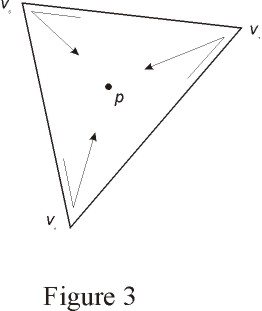## A Class to Represent Points

The representation for a point includes a pair of real numbers to store the x and y coordinates of the point (I use double), as well as member functions to read each coordinate (get_x and get_y). The basic arithmetic operations are provided in the form of overloaded operators. The arithmetic operations include addition, subtraction, and multiplication (scalar product). Also, multiplication and division by real numbers is provided in the form of overloaded operators.

The basic arithmetic operations allow you, for example, to obtain a point that is inside a triangle given its vertices as follows:

p = (v1 + v2 + v3) / 3;

Also, an overloaded member function is provided to test for inclusion in a triangle and in a polygon. This allows nice expressions, as shown below:

Point p; Triangle t; Polygon P; if (p.is_inside (t)) // ... if (p.is_inside (P)) // ...

Similarly, a function to test if a point is exactly on a segment is provided. This is determined by checking if the segment endpoints (p1,p2) and the point p are collinear, and if the segments p-p1 and p-p2 have opposite directions (which means that p is between p1 and p2).

Listings 1-a and 1-b show the code for the class definition and implementation (respectively) of class Point.

## A Class to Represent Segments

The class Segment contains, as data members, two Point objects that represent a non-oriented segment. (although in most algorithms we deal with it as an oriented segment from p1 to p2) No operators are provided for this class, since there are no natural arithmetic operations between segments.

A member function is provided to test for segment intersection. This member function allows expressions like the following:

Segment s, line; if (s.intersects (line)) // ...

Listings 2-a and 2-b show the code for the class interface and implementation of class Segment.

Both Point and Segment classes provide draw member functions. These member functions are platform dependent. I provide a simplified implementation for a Win32 platform (without scaling or other considerations). If you want to use these tools in an application that requires graphical display, you should provide the implementation for the draw member functions for Point and Segment.

## A Class to Represent Triangles

The class Triangle includes three Point objects representing the vertices of the triangle. It provides member functions to compute the area and the orientation. (a private member function is provided to compute the area with sign, which enables other member functions to obtain the area and the orientation of the triangle).

Since we are never interested in the exact value of the area of a triangle (at least not in this type of applications), the member function computes a value corresponding to twice the area of the triangle, for efficiency reasons. This may sound crazy, but you'll have to trust me on that one for now (the reasons should become clear later in this article).

Class Triangle also provides a member function to test if a point is inside the triangle. This member function allows expressions such as the following:

Triangle t; Point p; if (t.contains (p)) // ...

Note that the Point class member function to test for inclusion is equivalent to this Triangle class operation. The Point's member function is obviously implemented in terms of this Triangle's member function (see listing 1-b).

A member function draw is also included. In this case (as with the Polygon class, yet to be presented), the function is platform independent, since it is implemented in terms of the Segment class draw member function.

Listings 3-a and 3-b show the code for the class definition and implementation of class Triangle.

None of the above mentioned classes contains any pointers to dynamically allocated data or any allocated resources. Therefore, I provide no destructors, copy constructors or overloaded assignment operators for either of these classes.

## A Class to Represent Polygons

A circular doubly-linked list of Points is required to represent a polygon. The list must be doubly-linked because typical operations on polygons require insertions and removals of points in the sequence. Some of these operations must traverse the sequence in reverse, visiting more than one point in succession. The linked list must be circular because polygons are closed sequences of vertices, and operations on pairs or triplets of consecutive vertices may not be limited by the fact that a vertex is the last or the first in the chain.

The implementation of class Polygon uses the STL (Standard Template Library) list container. For convenience of use, I provide an iterator for this class, as the standard containers do. Thus, polygons can be manipulated in the same manner as most of the standard containers, as shown below:

Polygon P; Polygon::iterator current = P.begin(); do { // ... } while (++current != P.begin());

Notice that there is no end() member function for the Polygon container, since it is a circular list.

Also, the typical operations are similar to their equivalents for the standard containers (e.g., push_back, push_front, insert, remove), which should make polygon manipulation intuitive to C++ programmers.

No destructor, constructors or assignment operator are required for this class, since the container included as a data member encapsulates those operations.

Listings 4-a and 4-b show the definition and implementation of class Polygon and its iterators (class Polygon::iterator and class Polygon::const_iterator). Note that the implementation of these iterators is based on the list iterator. Their operations are almost a direct map to the operations of the list iterator, except for the ++ and -- operators, since the polygon is a circular list of points.

## Geometric Algorithms for Polygons

I will now describe a few algorithms related to polygons, and how I implemented them in class Polygon. Some of the algorithms are implemented as member functions, since they correspond to operations inherent to a polygon, and some are implemented as global functions that use the polygons and their related operations to perform the processing required.

Unless otherwise specified, polygons are assumed to be simple (i.e., no edges intersect, and no two vertices are the same point), and, when it is required to assume an orientation, it will be assumed that the vertices are oriented in counter-clockwise sense. When dealing with polygons, the term orientation refers to the apparent sense of rotation when traversing a polygon's vertices. In particular, if the orientation is counter-clockwise, then the interior of the polygon will always "touch" the edges on their left. This further assumes that we are dealing with edges as oriented segments from one vertex to the next vertex -- i.e., from Vn to Vn+1.

### Testing for Point Inclusion in a Polygon

To test if a point p is inside a polygon P, we need find a point that is outside the polygon, and count how many times the line L from p to the point outside intersects the polygon. To find a point outside the polygon, we obtain the vertex with highest x-coordinate and we add one to that x-coordinate.

 Each time that the line L intersects the polygon, it passes from inside to outside or vice versa. Thus, if the number of intersections is even, the point is outside the polygon. If it is odd, the point is inside. We only need to be careful when the line L passes exactly over a vertex of the polygon. In that case, we need to check if L intersects the polygon (figure 4-a) or if it merely touches it (figure 4-b). This is determined by checking if the segments around the intersecting vertex are on different sides of L. Furthermore, if several consecutive vertices are exactly on the line L, they have to be discarded before checking if L intersects or touches the polygon. (see listing 4-b)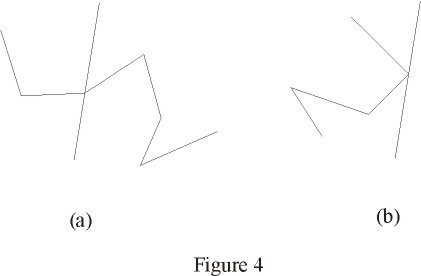### Testing for Polygon Orientation

The orientation of a polygon is often known in advance, since it may be guaranteed by the mechanism used to obtain the polygon. In some situations, though, we may have no more at our disposal than a sequence of points, with the knowledge that it represents a simple polygon. Determining the orientation of such a polygon consists of three steps:

1) Find a triplet of consecutive vertices such that the triangle formed by those three vertices does not contain any other vertex of the polygon. If no other vertex is inside, then the region inside that triangle is either entirely inside the polygon, or entirely outside the polygon.

2) Determine whether the interior of the triangle found in 1) lies inside or outside of the polygon. To do this, take any point inside the triangle and test if it is inside the polygon. If a point inside the triangle is also inside the polygon, the interior of the triangle lies inside the polygon as well. A point inside a triangle (p1,p2,p3), is given by (p1+p2+p3) / 3.

3) Determine the orientation of the triplet of points making up the triangle. If the triplet represents a left turn and the triangle is inside the polygon, then the polygon is counter-clockwise. Also, if the triplet represents a right turn and the triangle is outside the polygon, then the polygon is counter-clockwise. The other two combinations correspond to a polygon with clockwise orientation (see listing 4-b).

### Finding the Convex Hull of a Simple Polygon

The convex hull of a set R is defined as the smallest (in area) convex region that contains the entire set R, where R can be a set of points in the plane, a region, a polygon, the interior of a polygon, etc. If the set R is a set of points (i.e., a finite number of points) or a polygon, it can be easily proven that the convex hull is a convex polygon. Figure 5 shows a simple polygon outlined with solid lines, and its convex hull, in dashed lines.

For an arbitrary set of N points, it has been proven that the convex hull can not be obtained in less than O(N logN). If the points are known to form a simple polygon, the scenario is different. For a simple polygon, several algorithms have been proposed to find the convex hull in linear time (O(N) operations).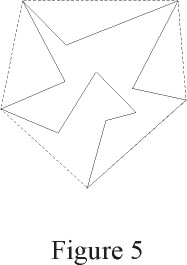Most of those algorithms have been proven to be incorrect, including the first O(N) algorithm proposed, known as the Sklansky scan (also known as the three coins algorithm), which was accepted and used for several years, until a counter-example was published, proving that the algorithm would work incorrectly for most arbitrary polygons.

A detailed discussion about the various convex hull algorithms would be beyond the scope of this article. I want only to make it clear that the problem is far more complicated than it looks, despite the simplicity of the algorithm that I present in this article (the Melkman algorithm).

Melkman proposed an extremely elegant and straightforward algorithm to compute (in linear time) the convex hull of a simple polygonal chain (which can obviously be used for a simple polygon). The key idea in this algorithm is to maintain an updated version of the convex hull, and for each new point encountered in the input polygonal chain, we test if the convex hull needs to be updated.

The output at any step of the algorithm is a convex polygon with counter-clockwise orientation, whose first and last elements are the same point, and which correspond to the last point appended to the output. Thus, the algorithm starts by sending to the output the first three points of the input polygon (with the appropriate orientation).

At the step n of the algorithm, we test if the new point (point number n+1 of the input polygon) is outside the convex hull. If it is, then the convex hull needs to be updated to account for the new point. If not, the new point is discarded and the output remains the same.

 Figure 6 shows an example of the output convex hull, showing the first two and the last two points, as well as several possibilities of location for the new point (Pn+1). Since the algorithm should work in linear time, the test to determine if the new point is inside the polygon must be done in constant time. Thus, we cannot use the function previously described to test for point inclusion in a polygon, since it does not operate in constant time. In this case, given the way we construct the current convex hull, Melkman showed a way to determine in constant time if the point is inside the current convex hull. If the new point is at the right of the oriented segment P2-P1 and at the left of the oriented segment Pn-1-Pn, then it is certain that the point is inside the convex hull, since the line or polyline going from Pn to Pn+1 (which is part of the input polygon) would have to intersect the convex hull for the point Pn+1 to be outside. If it intersects the convex hull, then it intersects the input polygon itself. But since the input polygon is simple, we know that there are no edge intersections.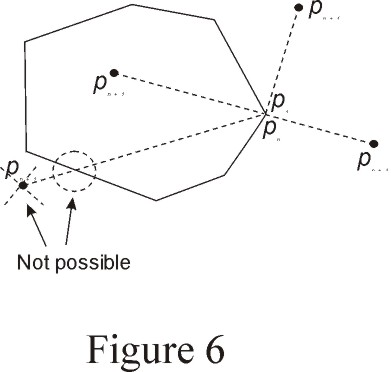Thus, by testing the orientation for these two triplets of points, we determine if the new point should be discarded or not. If it is inside, we discard it. If it is outside, then we append it at both ends of the output, and now we must readjust the output polygon by removing any vertices that became concave after the insertion of this new point.

All these updates involve only tests of orientation of triplets of points, since we know that the output polygon is in counter-clockwise sense, which means that a left turn represents a convex vertex, and a right turn represents a concave vertex.

 Figure 7 shows an example of this updating process. Notice that P1 and Pn are actually the same physical point. In the figure, I show them as two separate points only for illustration purposes.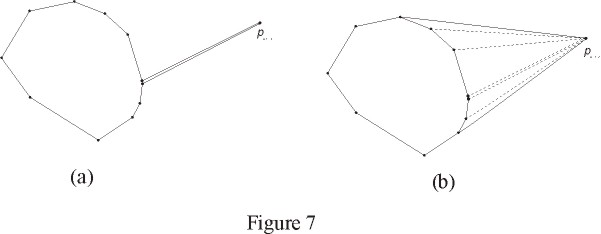Despite the fact that one step (after O(n) insertions) might involve O(n) removals, the algorithm does work in linear time (worst-case complexity O(N)). Each point from the input polygon can be inserted, at most, once into the output, and can be removed, at most, once. Therefore, we have, at most, 4*N operations (insertions are done at both ends of the output, and removals may be done from both ends). Since the test to decide if the point should be discarded is done in constant time, the algorithm works in linear time.

Listing 4-b shows the details of the implementation of this algorithm (it is implemented as a member function that returns a polygon without modifying the object that calls the member function).

### Triangulating a Polygon

Triangulation is an important and very useful operation in many geometry applications. It provides a representation of a complex figure in simpler pieces -- triangles.

The result of a triangulation process is a set of triangles for which each and every vertex can only be a vertex of the polygon being triangulated. Also, the triangles' internal regions must add up the entire interior of the polygon. (this is not a formal and rigorous definition of a triangulation, but you get the idea). Figure 8 shows an example of a polygon and a triangulation of it. Notice that for a given polygon, the triangulation is not necessarily unique.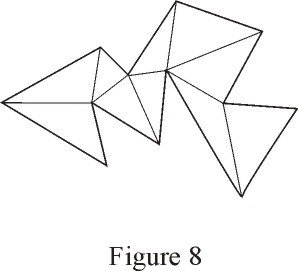Some applications require the output of a triangulation to be expressed as a graph in which adjacent triangles (triangles that share one edge) are adjacent in the graph. In this article, I restrict the scope of the triangulation algorithm to provide a non-ordered set of triangles.

The algorithm uses a divide-and-conquer recursive approach, which means that its expected complexity is N logN. Its worst case complexity, however, is O(N²).

The algorithm requires to know the orientation of the input polygon. Therefore, counter-clockwise orientation will be assumed. This allows to identify a convex vertex (when the triplet previous-current-next makes a left turn).

 The algorithm consists of finding convex vertices in the input polygon. For each convex vertex that we find, we consider the triangle Vprev-Vcurr-Vnext such that Vprev is the vertex previous to the convex vertex; Vcurr is the convex vertex; and Vnext is the point succeding the convex vertex. We now test if this triangle contains any other vertices of the input polygon. If the triangle does not contain any other vertex of the input polygon, then it is guaranteed that the segment Vprev,Vnext does not intersect any edge of the polygon, therefore, the triangle is sent to the output and the vertex Vcurr is removed from the polygon. If the triangle does contain other vertices of the input polygon, we find one of these vertices such that it can be joined to the vertex Vcurr without intersecting any edges of the polygon. If we call that vertex Vs, then the segment (Vcurr,Vs) divides the input polygon into two simple polygons. At this point, we recursively call the function to triangulate each half of the polygon. (see figure 9) The stop condition of the recursive process is, of course, when the input polygon has three vertices (i.e., when it is already a triangle).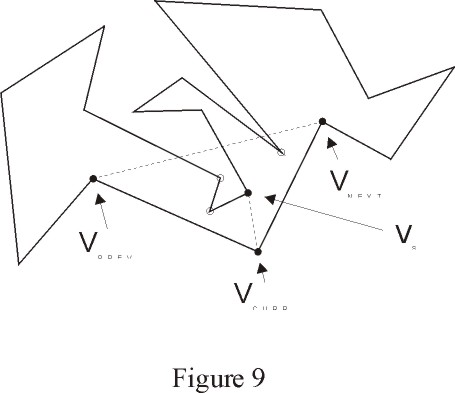The step of finding a vertex that can be joined to the vertex Vcurr in the triangle is not as obvious as it looks like. Many wrong approaches have been used in the past, including the most intuitive, which is finding the nearest vertex to Vcurr. Figure 10-a illustrates a situation in which this approach fails, since it produces an output with triangles that intersect the input polygon. The appropriate way to find such vertex is to find the point with highest perpendicular distance to the straight line containing the segment Vprev, Vnext, as illustrated in figure 10-b.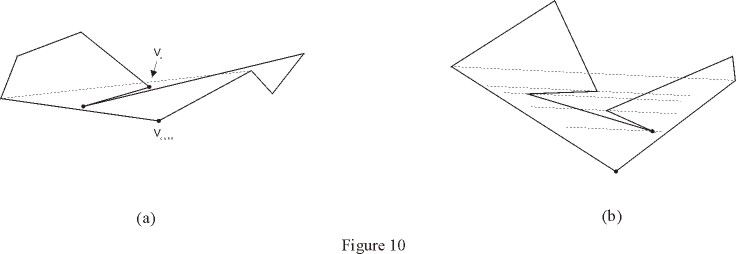Naturally, to obtain this point the algorithm does not directly compute a single distance (it would be too hard, since we are talking about perpendicular distance). Instead, the algorithm finds the point P for which the area of the triangle (Vprev,Vnext,P) is maximized. This area is equal to the base (which is fixed, since it is the distance between Vprev and Vnext) times the height (which is the perpendicular distance to the base segment) divided by two. Notice that we don't care that the member function area() actually returns twice the area: we only want to compare and find the highest area -- the actual value of the area is of no interest here.

Listing 5 show the code for this triangulation algorithm.

### Finding the Convex Hull of an Arbitrary Set of Points

Obviously, an approach to do this in O(N logN) would be to sort the points by their x-coordinate, which allows us to build a simple polygonal chain (there can not be edge intersections, since the points are sorted by their x-coordinate) and now use a linear time algorithm to obtain the convex hull.

A much more straightforward approach, known as the Jarvi's March, is the following: we first find a point that is on the convex hull (for example, the point with lowest y coordinate). This can be done in linear time. After that, we find the right-most point with respect to that first point. The right-most point with respect to point P is the point X for which no other point of the set is at the right of the oriented segment from P to X. The right-most point is also on the convex hull, so we append it to the output and advance. That is, we continue to find the right-most point with respect to the last point added. We keep going until the right-most point found is the first point.

Notice that finding the right-most point does not require any single angle calculation or manipulation -- it can be done by computing the orientations ofpoint triplets. Listing 6 shows the code for this algorithm.

## Conclusion

Geometric algorithms and techniques are important and convenient tools in many applications, including Image Processing, Graphics and Pattern Recognition.

Efficiency is in general a very important (maybe the most important) issue, since applications that involve this type of processing in general act on huge amounts of information (we may be talking about polygons with several hundreds or several thousands of vertices). In addition to that, this type of applications often require real-time processing, with very demanding timing restrictions.

The approach that I presented in this article is based on very efficient mathematical tools. Most of the operations required for standard geometric algorithms would not require any single computation of trigonometric functions, square roots, etc. Instead, a reduced number of multiplications and additions are required per item processed. In some cases, I sacrificed at some extent efficiency in exchange for "straightforwardness" of the code. I did this only in situations where I considered that the impact on the overall speed of execution is not considerable.

The Object Oriented approach turns out to be extremely appropriate for this application. Not only is it very intuitive to see geometric entities as software objects, but also, the approach leads to expressions that are very intuitive and readable. Also, C++ compilers in general provide very efficient machine code, which is, as I mentioned before, one of the most important aspects in this type of applications.

## Bibliography

Bjarne Stroustrup. The C++ Programming Language, 3rd Edition.